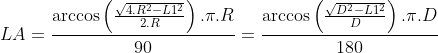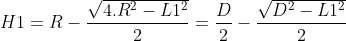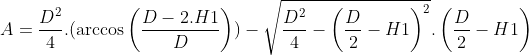# Circle sector calculator, formulas, practical use.

## Circle sector calculations

The circle sector calculator is designed for calculations radius and diameter of a circle sector, circumference and area of a circle sector, chord and arc length of a circle sector and height of a circle sector. For successful calculation, you need to know and enter into the calculator radius or diameter and at least one of the other valuesThen press send or enter to run calculations.

## Circle sector parameters sketch:﻿
Unit system:
•

•

•          °

•

•

## Circle sector calculator variables and constants explanation:

d – diameter
C – circumference
A – area
LA – arc length
π– 3,1415926535
β– sector angle
– unspecified values
specified values
calculated values

## The formula for circle arc length calculation:## The formula for circle arc length calculation:## The formula for circle chord length calculation:## The formula for circle segment height calculation:## The formula for circle segment area calculation:## The formula for circle segment area calculation:## The formula for circle segment circumference calculation:## The formula for circle segment area calculation:## Circle segment calculator use:

Calculations of circle segment for:

• technical calculation of D-shaped trimmed shaft in engineering industry
• area and circumference of a cross-section truncated cylinder
• school calculations of a truncated cylinder